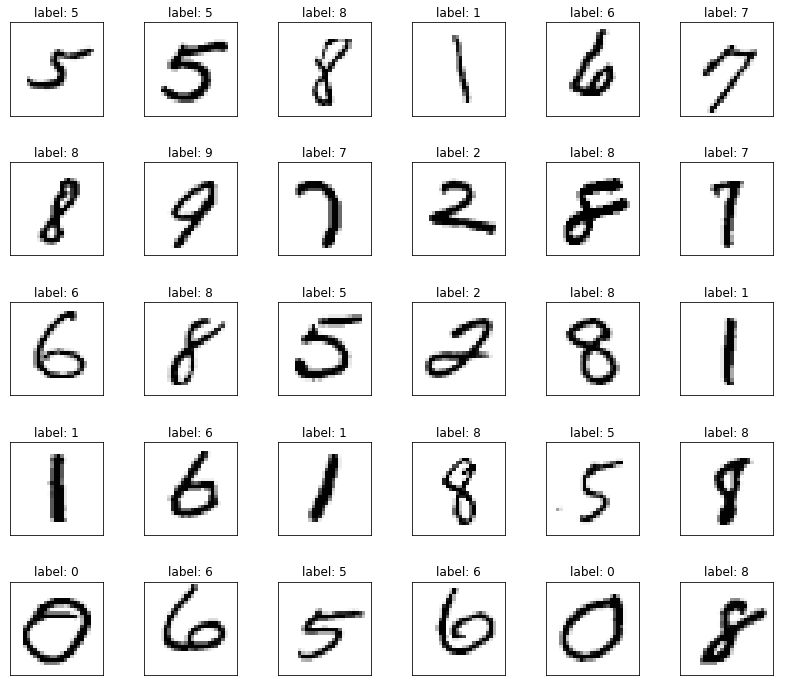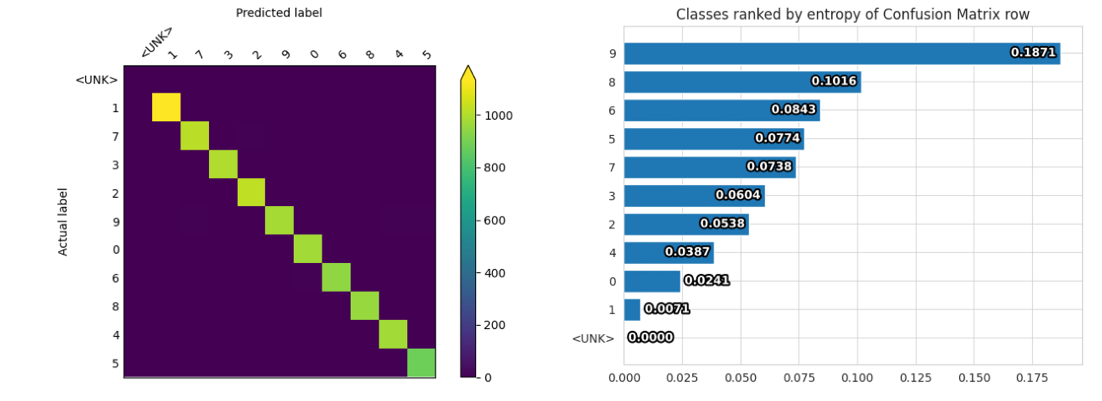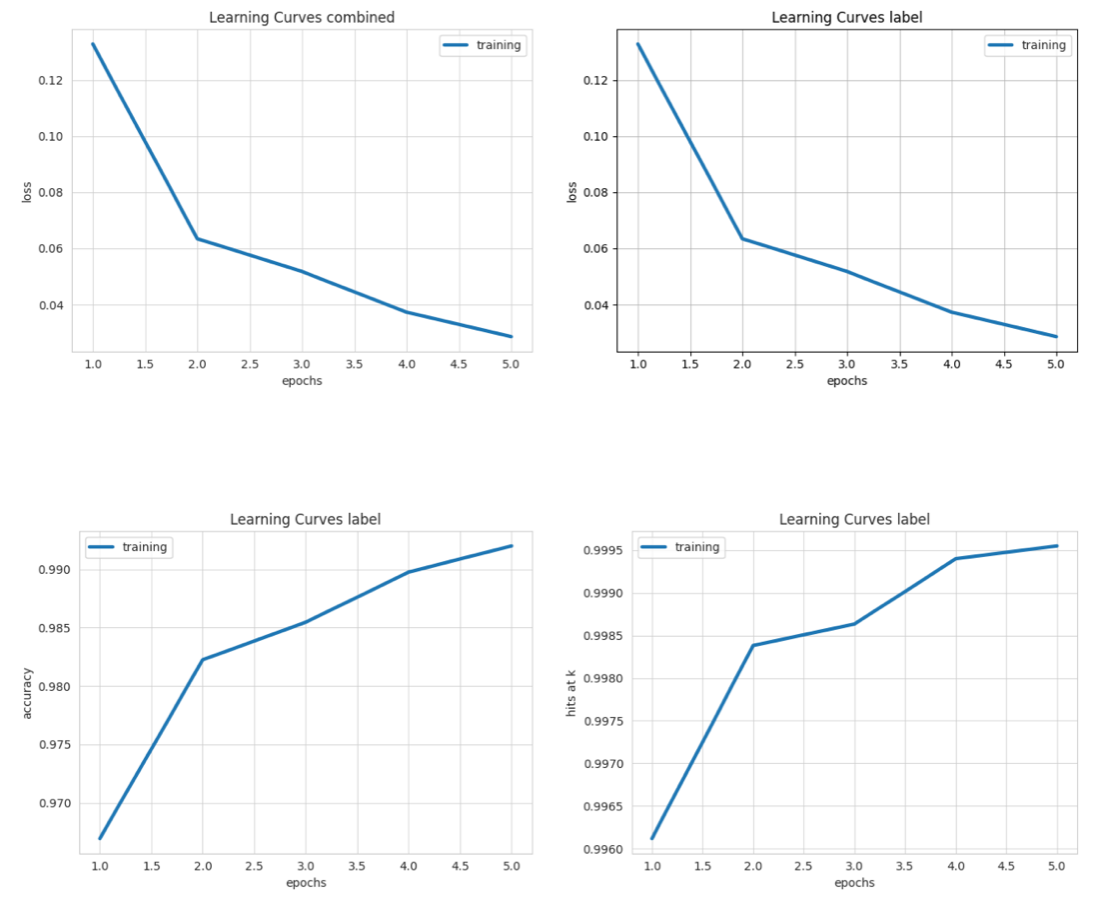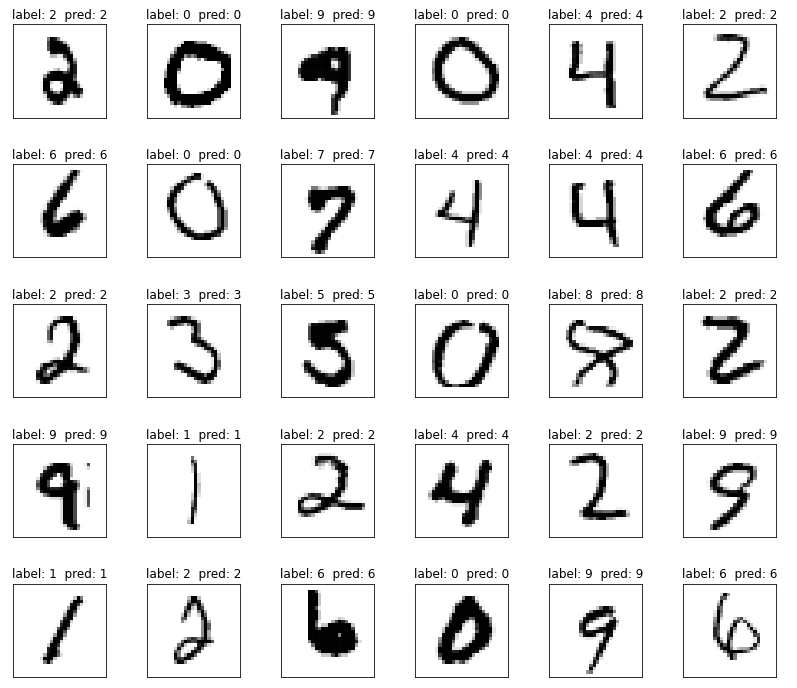# Image Classification

This is a complete example of training an image classification model on the MNIST handwritten digit dataset.

These interactive notebooks follow the steps of this example:

MNIST is a collection of gray-scale images of handwritten digits. This collection is made up of 60,000 images for training and 10,000 images for testing model performance. Each image is 28 X 28 pixels in gray-scale.

``````ludwig datasets download mnist
``````

This command will create a dataset `mnist_dataset.csv` in the current directory. In addition, there will be directories `training/` and `testing/` containing the images.

The columns in the dataset are

column description
image_path file path string for the image
label single digit 0 to 9 indicating what digit is shown in the image
split integer value indicating a training example (0) or test example (2)

``````from ludwig.datasets import mnist

# Loads the dataset as a pandas.DataFrame
``````
This will create two pandas DataFrames. `train_df` contains file path information to the 60K training images. `test_df` has same information for the 10K test images.

column description
image_path file path string for the image
label single digit 0 to 9 indicating what digit is shown in the image

Sample of images with `label`.### Train¶

The Ludwig configuration file describes the machine learning task. This example only uses a small subset of the options provided by Ludwig. Please refer to the Configuration Section for all the details.

First it defines the `input_features`. For the image feature, the configuration specifies the type of neural network architecture to encode the image. In this example the encoder is a two layer Stacked Convolutional Neural Network followed by a fully connected layer with dropout regularization.

Next the `output_features` are defined. In this case, there is only one output feature called `label`. This is a categorical feature that indicates the digit the image represents, 0, 1, 2, ..., 9.

The last section in this configuration file describes options for how the the `trainer` will operate. In this example the `trainer` will process the training data for 5 epochs.

``````# config.yaml
input_features:
- name: image_path
type: image
encoder:
type: stacked_cnn
conv_layers:
- num_filters: 32
filter_size: 3
pool_size: 2
pool_stride: 2
- num_filters: 64
filter_size: 3
pool_size: 2
pool_stride: 2
dropout: 0.4
fc_layers:
- output_size: 128
dropout: 0.4

output_features:
- name: label
type: category

trainer:
epochs: 5
``````

LudwigModel

``````# create Ludwig configuration dictionary
config = {
'input_features': [
{
'name': 'image_path',
'type': 'image',
'preprocessing': {'num_processes': 4},
'encoder': {
'stacked_cnn',
'conv_layers': [
{'num_filters': 32, 'filter_size': 3, 'pool_size': 2, 'pool_stride': 2},
{'num_filters': 64, 'filter_size': 3, 'pool_size': 2, 'pool_stride': 2, 'dropout': 0.4}
],
'fc_layers': [{'output_size': 128, 'dropout': 0.4}]
}
}
],
'output_features': [{'name': 'label', 'type': 'category'}],
'trainer': {'epochs': 5}
}

# Constructs Ludwig model from config dictionary
model = LudwigModel(config, logging_level=logging.INFO)
``````

Train the model.

`ludwig train` command

``````ludwig train \
--dataset mnist_dataset.csv \
--config config.yaml
``````

train() method

``````# Trains the model. This cell might take a few minutes.
train_stats, preprocessed_data, output_directory = model.train(dataset=train_df)
``````

### Evaluate¶

Evaluate the trained model.

`ludwig evaluate` command

``````ludwig evaluate --model_path results/experiment_run/model \
--dataset mnist_dataset.csv \
--split test \
--output_directory test_results
``````

evaluate() method

``````# Generates predictions and performance statistics for the test set.
test_stats, predictions, output_directory = model.evaluate(
test_df,
collect_predictions=True,
collect_overall_stats=True
)
``````

### Visualize Metrics¶

Display Confusion Matrix and Class Entropy plots.

`ludwig visualize confusion_matrix` command

``````ludwig visualize --visualization confusion_matrix \
--test_statistics test_results/test_statistics.json \
--output_directory visualizations \
--file_format png
``````

`visualize.confusion_matrix()` function

``````# Visualizes confusion matrix, which gives an overview of classifier performance
# for each class.
from ludwig.visualize import confusion_matrix

confusion_matrix(
[test_stats],
'label',
top_n_classes=,
model_names=[''],
normalize=True,
)
``````Display Learning Curves plots.

`ludwig visualize learning_curves` command

``````ludwig visualize --visualization learning_curves \
--training_statistics results/experiment_run/training_statistics.json \
--file_format png \
--output_directory visualizations
``````

`visualize.learning_curves()` function

``````# Visualizes learning curves, which show how performance metrics changed over
# time during training.
from ludwig.visualize import learning_curves

learning_curves(train_stats, output_feature_name='label')
``````### Predictions¶

Generate predictions from test dataset.

`ludwig predict` command

``````ludwig predict --model_path results/experiment_run/model \
--dataset mnist_dataset.csv \
--split test \
--output_directory predictions
``````

`predict()` method

``````predictions, output_directory = model.predict(test_df)
``````

Sample test images displaying true("label") and predicted("pred") labels.